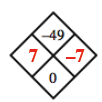### Home > CC2MN > Chapter 6 Unit 6A > Lesson CC3: 6.2.4 > Problem6-85

6-85.

Copy and complete each of the Diamond Problems below. The pattern used in the Diamond Problems is shown at right.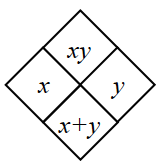1.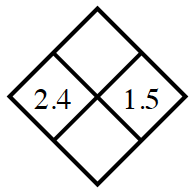What are the product and sum of $2.4$ and $1.5$?

1.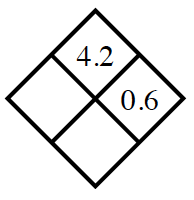$?(0.6)=4.2$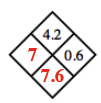1.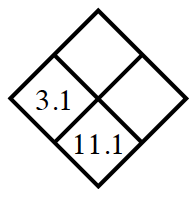$3.1+?=11.1$

1.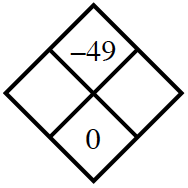Which two numbers have a product of $-49$ and a sum of $0$?

Since the sum is $0$, one number must be negative and one must be positive, but both numbers must have the same magnitude.